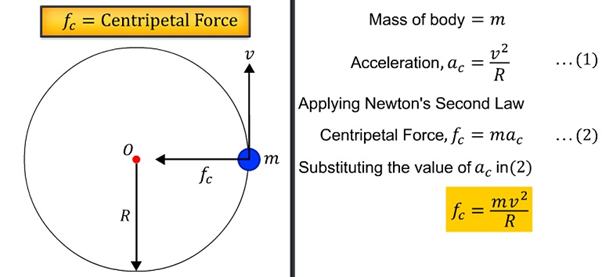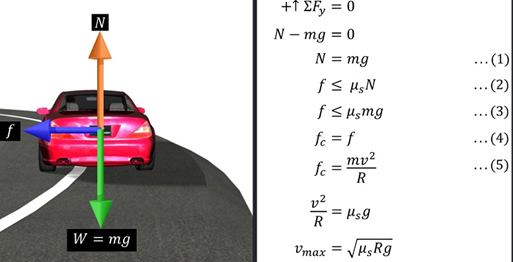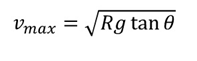Notes On Circular Motion and Banking - CBSE Class 11 Physics
When an object is in uniform circular motion, the force directed towards the centre is called the centripetal force.In the case of  a stone tied to a string and rotated in a circle in a horizontal plane, the tension, T, in the string provides the required centripetal force. This tension in the string is due to the pull exerted on the string by the person, which prevents the stone from flying away. Similarly, a car moving along a circular path at uniform speed also requires a centripetal force to keep it moving along the desired circular path.   An object moving along a circular path at uniform speed also requires a centripetal force to keep it moving along the desired circular path. Let us consider a car moving on a level, curved road. There are three forces acting on the car, namely: weight of the car, mg, normal reaction, N, and frictional force, f.Now let us consider a car moving on a banked road. The weight of the car, mg, acts vertically downwards. The normal reaction, N, acts upwards perpendicular to the road’s surface. The impending motion of the car in the lateral direction is away from the car in the lateral direction. The frictional force, f, acts opposite to this direction, that is, down the lateral slope.If the frictional force is negligible, the maximum velocity vmax can be shown to beIf the speed of the car is more than vmax it starts kidding and moves away from the circular track.

#### Summary

When an object is in uniform circular motion, the force directed towards the centre is called the centripetal force.In the case of  a stone tied to a string and rotated in a circle in a horizontal plane, the tension, T, in the string provides the required centripetal force. This tension in the string is due to the pull exerted on the string by the person, which prevents the stone from flying away. Similarly, a car moving along a circular path at uniform speed also requires a centripetal force to keep it moving along the desired circular path.   An object moving along a circular path at uniform speed also requires a centripetal force to keep it moving along the desired circular path. Let us consider a car moving on a level, curved road. There are three forces acting on the car, namely: weight of the car, mg, normal reaction, N, and frictional force, f.Now let us consider a car moving on a banked road. The weight of the car, mg, acts vertically downwards. The normal reaction, N, acts upwards perpendicular to the road’s surface. The impending motion of the car in the lateral direction is away from the car in the lateral direction. The frictional force, f, acts opposite to this direction, that is, down the lateral slope.If the frictional force is negligible, the maximum velocity vmax can be shown to beIf the speed of the car is more than vmax it starts kidding and moves away from the circular track.

Previous
Next